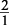## Geometric Progressions

A geometric progression is a progression in which there is a constant ratio between terms. The value found by dividing any term by the term before it gives you the constant ratio.

##### Example 1

What is the next number in the progression 1, 2, 4, 8, 16, … ?

The ratio is =because. You could also have said that you're simply doubling each number to get the next number. So doubling the last number, 16, gives 32.

##### Example 2

What is the next number in the progression 3, 9, 27, 81, … ?

The ratio isbecause. You could also have said you are multiplying each number by 3 to get the next number. So multiply the last number, 81, by 3, which gives 243.